# Image Transmission and Reception Using 802.11 Waveform and SDR

This example shows how to encode and pack an image file into WLAN packets for transmission and subsequently decode the packets to retrieve the image. The example also shows how to use a software-defined radio (SDR) for over-the-air transmission and reception of the WLAN packets.

### Introduction

This example imports and segments an image file into multiple medium access control (MAC) service data units (MSDUs). It passes each MSDU to the `wlanMACFrame` function to create a MAC protocol data unit (MPDU). This function also utilizes a `wlanMACFrameConfig` object as an input, which sequentially numbers the MPDUs through the `SequenceNumber` property. The example then passes the MPDUs to the physical (PHY) layer as PHY Layer Service Data Units (PSDUs). Each PSDU uses a single non-high-throughput (non-HT), 802.11a™ WLAN packet for transmission. This example creates a WLAN baseband waveform using the `wlanWaveformGenerator` function. This function utilizes multiple PSDUs and processes each to form a series of physical layer convergence procedure (PLCP) protocol data units (PPDUs) ready for transmission.The generated waveform then passes through an additive white Gaussian noise (AWGN) channel to simulate an over-the-air transmission. Subsequently, the `wlanMPDUDecode` function takes the noisy waveform and decodes it. Then, the `SequenceNumber` property in the recovered MAC frame configuration object allows the example to sequentially order the extracted MSDUs. The information bits in the multiple received MSDUs combine to recover the transmitted image. This diagram shows the receiver processing.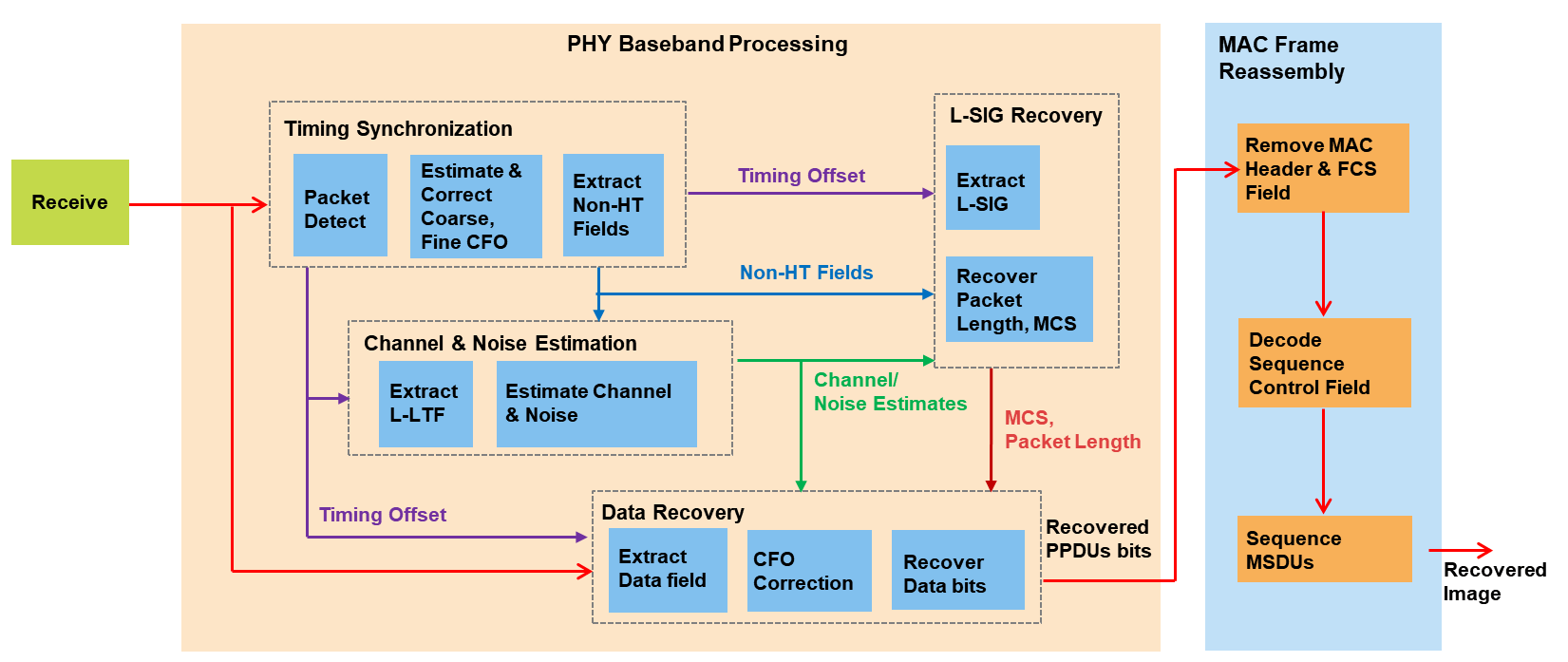Alternatively, the generated WLAN waveform can be transmitted over the air and received using these supported SDRs.Communications Toolbox Support Package for USRP® Embedded Series Radio

Communications Toolbox Support Package for Xilinx® Zynq®-Based Radio

### Example Setup

Before running the example, set the `channel` variable to be one of these options:

• `OverTheAir`: Use an SDR to transmit and receive the WLAN waveform

• `GaussianNoise`: Pass the transmission waveform through an AWGN channel (default)

• `NoImpairments`: Pass the transmission waveform through with no impairments

If you set `channel` to `OverTheAir`, set frequency band and channel, transmission gain, reception gain, and desired SDR:

• Set to `Pluto` to use the ADALM-Pluto Radio (default)

• Set to `E3xx` to use the USRP Embedded Series Radio

• Set to `AD936x` or `FMCOMMS5` to use the Xilinx Zynq-Based Radio

```channel ="GaussianNoise"; if strcmpi(channel,"OverTheAir") deviceName =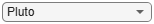"Pluto"; channelNumber =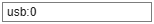5; frequencyBand =2.4; txGain =-10; rxGain =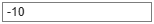10; elseif strcmpi(channel,"GaussianNoise") % Specify SNR of received signal for a simulated channel SNR =20; end```

Configure all the scopes and figures for the example.

```% Setup handle for image plot if ~exist('imFig','var') || ~ishandle(imFig) %#ok<SUSENS> imFig = figure; imFig.NumberTitle = 'off'; imFig.Name = 'Image Plot'; imFig.Visible = 'off'; else clf(imFig); % Clear figure imFig.Visible = 'off'; end % Setup Spectrum viewer spectrumScope = spectrumAnalyzer( ... SpectrumType='power-density', ... Title='Received Baseband WLAN Signal Spectrum', ... YLabel='Power spectral density', ... Position=[69 376 800 450]); % Setup the constellation diagram viewer for equalized WLAN symbols refQAM = wlanReferenceSymbols('64QAM'); constellation = comm.ConstellationDiagram(... Title='Equalized WLAN Symbols',... ShowReferenceConstellation=true,... ReferenceConstellation=refQAM,... Position=[878 376 460 460]);```

### Transmitter Design

These steps describe the general procedure of the WLAN transmitter.

1. Import an image file and convert it to a stream of decimal bytes

2. Generate a baseband WLAN signal using the WLAN Toolbox

3. Pack the data stream into multiple 802.11a packets

If using an SDR, these steps describe the setup of the SDR transmitter.

1. Prepare the baseband signal for transmission using the SDR hardware

2. Send the baseband data to the SDR hardware for upsampling and continuous transmission at the desired center frequency

Prepare Image File

Read data from the image file, scale it for transmission, and convert it to a stream of decimal bytes. The scaling of the image reduces the quality by decreasing the size of the binary data stream.

The size of the binary data stream impacts the number of WLAN packets required for the transmission of the image data. The number of WLAN packets generated for transmission depends on these factors.

1. The image scaling, set when importing the image file

2. The length of the data carried in a packet, specified by the `msduLength` variable

3. The modulation and coding scheme (MCS) value of the transmitted packet

The combination of the scaling factor and MSDU length determines the number of WLAN radio packets required for transmission. Setting `scale` to `0.2` and `msduLength` to `2304` requires the transmission of 11 WLAN radio packets. Increasing the scaling factor or decreasing the MSDU length will result in the transmission of more packets.

```% Input an image file and convert to binary stream fileTx =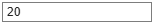'peppers.png'; % Image file name fData = imread(fileTx); % Read image data from file scale =0.2; % Image scaling factor origSize = size(fData); % Original input image size scaledSize = max(floor(scale.*origSize(1:2)),1); % Calculate new image size heightIx = min(round(((1:scaledSize(1))-0.5)./scale+0.5),origSize(1)); widthIx = min(round(((1:scaledSize(2))-0.5)./scale+0.5),origSize(2)); fData = fData(heightIx,widthIx,:); % Resize image imsize = size(fData); % Store new image size txImage = fData(:); % Plot transmit image imFig.Visible = 'on'; subplot(211); imshow(fData); title('Transmitted Image'); subplot(212); title('Received image appears here...'); set(gca,'Visible','off');````set(findall(gca, 'type', 'text'), 'visible', 'on');`

Fragment Transmit Data

Split the data stream (`txImage`) into smaller transmit units (MSDUs) of size `msduLength`. Then, create an MPDU for each transmit unit using the `wlanMACFrame` function. Each call to this function creates an MPDU corresponding to the given MSDU and the frame configuration object. Next, create the frame configuration object using `wlanMACFrameConfig` to configure the sequence number of the MPDU. All the MPDUs are then sequentially passed to the physical layer for transmission.

To ensure that the MSDU size of the transmission does not exceed the standard-specified maximum, set the `msduLength` field to `2304` bytes. To make all MPDUs the same size, append zeros to the data in the last MPDU.

```msduLength =2304; % MSDU length in bytes numMSDUs = ceil(length(txImage)/msduLength); padZeros = msduLength-mod(length(txImage),msduLength); txData = [txImage;zeros(padZeros,1)]; txDataBits = double(int2bit(txData,8,false)); % Divide input data stream into fragments bitsPerOctet = 8; data = zeros(0,1); for i=0:numMSDUs-1 % Extract image data (in octets) for each MPDU frameBody = txData(i*msduLength+1:msduLength*(i+1),:); % Create MAC frame configuration object and configure sequence number cfgMAC = wlanMACFrameConfig(FrameType='Data',SequenceNumber=i); % Generate MPDU [psdu, lengthMPDU]= wlanMACFrame(frameBody,cfgMAC,OutputFormat='bits'); % Concatenate PSDUs for waveform generation data = [data; psdu]; %#ok<AGROW> end```

Generate 802.11a Baseband WLAN Signal

Synthesize a non-HT waveform using the `wlanWaveformGenerator` function with a non-HT format configuration object created by the `wlanNonHTConfig` object. In this example, the configuration object has a 20 MHz bandwidth, one transmit antenna and 64QAM rate 2/3 (MCS 6).

```nonHTcfg = wlanNonHTConfig; % Create packet configuration nonHTcfg.MCS = 6; % Modulation: 64QAM Rate: 2/3 nonHTcfg.NumTransmitAntennas = 1; % Number of transmit antenna chanBW = nonHTcfg.ChannelBandwidth; nonHTcfg.PSDULength = lengthMPDU; % Set the PSDU length```

Initialize the scrambler with a random integer for each packet.

`scramblerInitialization = randi([1 127],numMSDUs,1);`

Set the oversampling factor to `1.5` to generate the waveform at 30 MHz for transmission.

```osf =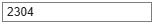1.5; sampleRate = wlanSampleRate(nonHTcfg); % Nominal sample rate in Hz % Generate baseband NonHT packets separated by idle time txWaveform = wlanWaveformGenerator(data,nonHTcfg, ... NumPackets=numMSDUs,IdleTime=20e-6, ... ScramblerInitialization=scramblerInitialization,... OversamplingFactor=osf);```

Configure SDR for Transmission

If using an SDR, set the transmitter gain parameter (`txGain`) to reduce transmission quality and impair the received waveform.

Create an `sdrTransmitter` object using the `sdrtx` function. Set the center frequency, sample rate, and gain to the corresponding properties of the `sdrTransmitter` object. For an 802.11a signal on channel 5 in the 2.4 GHz frequency band, the corresponding center frequency is 2.432 GHz as defined in section 16.3.6.3 of the IEEE Std 802.11-2016.

The `sdrTransmitter` object uses the transmit repeat functionality to transmit the baseband WLAN waveform in a loop from the double data rate (DDR) memory on the SDR.

```if strcmpi(channel,"OverTheAir") % Transmitter properties sdrTransmitter = sdrtx(deviceName); sdrTransmitter.BasebandSampleRate = sampleRate*osf; sdrTransmitter.CenterFrequency = wlanChannelFrequency(channelNumber,frequencyBand); sdrTransmitter.Gain = txGain; % Pass the SDR I/O directly to host skipping FPGA on Zynq Radio or USRP % Embedded Series Radio if ~strcmpi(deviceName,"Pluto") sdrTransmitter.ShowAdvancedProperties = true; sdrTransmitter.BypassUserLogic = true; end fprintf('\nGenerating WLAN transmit waveform:\n') % Scale the normalized signal to avoid saturation of RF stages powerScaleFactor = 0.8; txWaveform = txWaveform.*(1/max(abs(txWaveform))*powerScaleFactor); % Transmit RF waveform transmitRepeat(sdrTransmitter,txWaveform); end```

The `transmitRepeat` function transfers the baseband WLAN packets with idle time to the SDR, and stores the signal samples in hardware memory. The example then transmits the waveform continuously over the air until the release of the transmit object.

The steps listed below describe the general structure of the WLAN receiver.

1. If using SDR hardware, capture multiple packets of the transmitted WLAN signal

2. Detect a packet

3. Coarse carrier frequency offset is estimated and corrected

4. Fine timing synchronization is established. The L-STF, L-LTF and L-SIG samples are provided for fine timing to allow to adjust the packet detection at the start or end of the L-STF

5. Fine carrier frequency offset is estimated and corrected

6. Perform a channel estimation for the received signal using the L-LTF

7. Detect the format of the packet

8. Decode the L-SIG field to recover the MCS value and the length of the data portion

9. Decode the data field to obtain the transmitted data within each packet

10. Decode the received PSDU and check if the frame check sequence (FCS) passed for the PSDU

11. Order the decoded MSDUs based on the `SequenceNumber` property in the recovered MAC frame configuration object

12. Combine the decoded MSDUs from all the transmitted packets to form the received image

This example plots the power spectral density (PSD) of the received waveform, and shows visualizations of the equalized data symbols and the received image.

If using an SDR, create an `sdrReceiver` object using the `sdrrx` function. Set the center frequency, sample rate, and output data type to the corresponding properties of the `sdrReceiver` object.

Otherwise, apply gaussian noise to the `txWaveform` using the `awgn` function or pass the `txWaveform` straight through to receiver processing.

```if strcmpi(channel,"OverTheAir") sdrReceiver = sdrrx(deviceName); sdrReceiver.BasebandSampleRate = sdrTransmitter.BasebandSampleRate; sdrReceiver.CenterFrequency = sdrTransmitter.CenterFrequency; sdrReceiver.OutputDataType = 'double'; sdrReceiver.GainSource = 'Manual'; sdrReceiver.Gain = rxGain; if ~strcmpi(deviceName,"Pluto") sdrReceiver.ShowAdvancedProperties = true; sdrReceiver.BypassUserLogic = true; end % Configure the capture length equivalent to twice the length of the % transmitted signal, this is to ensure that PSDUs are received in order. % On reception the duplicate MAC fragments are removed. sdrReceiver.SamplesPerFrame = 2*length(txWaveform); fprintf('\nStarting a new RF capture.\n') rxWaveform = capture(sdrReceiver,sdrReceiver.SamplesPerFrame,'Samples'); elseif strcmpi(channel,"GaussianNoise") rxWaveform = awgn(txWaveform,SNR,'measured'); else % No Impairments rxWaveform = txWaveform; end```

Show the power spectral density of the received waveform.

```spectrumScope.SampleRate = sampleRate*osf; spectrumScope(rxWaveform); release(spectrumScope);```Design a rate conversion filter for resampling the waveform to the nominal baseband rate for receiver processing using the `designMultirateFIR` function.

```aStop = 40; % Stopband attenuation ofdmInfo = wlanNonHTOFDMInfo('NonHT-Data',nonHTcfg); % OFDM parameters SCS = sampleRate/ofdmInfo.FFTLength; % Subcarrier spacing txbw = max(abs(ofdmInfo.ActiveFrequencyIndices))*2*SCS; % Occupied bandwidth [L,M] = rat(1/osf); maxLM = max([L M]); R = (sampleRate-txbw)/sampleRate; TW = 2*R/maxLM; % Transition width b = designMultirateFIR(L,M,TW,aStop);```

Resample the oversampled waveform back to 20 MHz for processing using the `dsp.FIRRateConverter` System object and the filter designed above.

```firrc = dsp.FIRRateConverter(L,M,b); rxWaveform = firrc(rxWaveform);```

If using an SDR, the SDR continuously transmits the 802.11 waveform over-the-air in a loop. The first packet received by the `sdrReceiver` may not be the first transmitted packet. This means that the packets may be decoded out of sequence. To enable the received packets to be recombined in the correct order, their sequence number must be determined. The `wlanMPDUDecode` function decodes the MPDU from the decoded PSDU bits of each packet and outputs the MSDU as well as the recovered MAC frame configuration object `wlanMACFrameConfig`. The `SequenceNumber` property in the recovered MAC frame configuration object can be used for ordering the MSDUs in the transmitted sequence.

To display each packet's decoded L-SIG contents, the EVM measurements, and sequence number, check the `displayFlag` box.

`displayFlag =false; `

Set up required variables for receiver processing.

```rxWaveformLen = size(rxWaveform,1); searchOffset = 0; % Offset from start of the waveform in samples```

Get the required field indices within a PSDU.

```ind = wlanFieldIndices(nonHTcfg); Ns = ind.LSIG(2)-ind.LSIG(1)+1; % Number of samples in an OFDM symbol % Minimum packet length is 10 OFDM symbols lstfLen = double(ind.LSTF(2)); % Number of samples in L-STF minPktLen = lstfLen*5; pktInd = 1; fineTimingOffset = []; packetSeq = []; rxBit = []; % Perform EVM calculation evmCalculator = comm.EVM(AveragingDimensions=[1 2 3]); evmCalculator.MaximumEVMOutputPort = true;```

Use a `while` loop to process the received out-of-order packets.

```while (searchOffset+minPktLen)<=rxWaveformLen % Packet detect pktOffset = wlanPacketDetect(rxWaveform,chanBW,searchOffset,0.5); % Adjust packet offset pktOffset = searchOffset+pktOffset; if isempty(pktOffset) || (pktOffset+double(ind.LSIG(2))>rxWaveformLen) if pktInd==1 disp('** No packet detected **'); end break; end % Extract non-HT fields and perform coarse frequency offset correction % to allow for reliable symbol timing nonHT = rxWaveform(pktOffset+(ind.LSTF(1):ind.LSIG(2)),:); coarseFreqOffset = wlanCoarseCFOEstimate(nonHT,chanBW); nonHT = frequencyOffset(nonHT,sampleRate,-coarseFreqOffset); % Symbol timing synchronization fineTimingOffset = wlanSymbolTimingEstimate(nonHT,chanBW); % Adjust packet offset pktOffset = pktOffset+fineTimingOffset; % Timing synchronization complete: Packet detected and synchronized % Extract the non-HT preamble field after synchronization and % perform frequency correction if (pktOffset<0) || ((pktOffset+minPktLen)>rxWaveformLen) searchOffset = pktOffset+1.5*lstfLen; continue; end fprintf('\nPacket-%d detected at index %d\n',pktInd,pktOffset+1); % Extract first 7 OFDM symbols worth of data for format detection and % L-SIG decoding nonHT = rxWaveform(pktOffset+(1:7*Ns),:); nonHT = frequencyOffset(nonHT,sampleRate,-coarseFreqOffset); % Perform fine frequency offset correction on the synchronized and % coarse corrected preamble fields lltf = nonHT(ind.LLTF(1):ind.LLTF(2),:); % Extract L-LTF fineFreqOffset = wlanFineCFOEstimate(lltf,chanBW); nonHT = frequencyOffset(nonHT,sampleRate,-fineFreqOffset); cfoCorrection = coarseFreqOffset+fineFreqOffset; % Total CFO % Channel estimation using L-LTF lltf = nonHT(ind.LLTF(1):ind.LLTF(2),:); demodLLTF = wlanLLTFDemodulate(lltf,chanBW); chanEstLLTF = wlanLLTFChannelEstimate(demodLLTF,chanBW); % Noise estimation noiseVarNonHT = wlanLLTFNoiseEstimate(demodLLTF); % Packet format detection using the 3 OFDM symbols immediately % following the L-LTF format = wlanFormatDetect(nonHT(ind.LLTF(2)+(1:3*Ns),:), ... chanEstLLTF,noiseVarNonHT,chanBW); disp([' ' format ' format detected']); if ~strcmp(format,'Non-HT') fprintf(' A format other than Non-HT has been detected\n'); searchOffset = pktOffset+1.5*lstfLen; continue; end % Recover L-SIG field bits [recLSIGBits,failCheck] = wlanLSIGRecover( ... nonHT(ind.LSIG(1):ind.LSIG(2),:), ... chanEstLLTF,noiseVarNonHT,chanBW); if failCheck fprintf(' L-SIG check fail \n'); searchOffset = pktOffset+1.5*lstfLen; continue; else fprintf(' L-SIG check pass \n'); end % Retrieve packet parameters based on decoded L-SIG [lsigMCS,lsigLen,rxSamples] = helperInterpretLSIG(recLSIGBits,sampleRate); if (rxSamples+pktOffset)>length(rxWaveform) disp('** Not enough samples to decode packet **'); break; end % Apply CFO correction to the entire packet rxWaveform(pktOffset+(1:rxSamples),:) = frequencyOffset(... rxWaveform(pktOffset+(1:rxSamples),:),sampleRate,-cfoCorrection); % Create a receive Non-HT config object rxNonHTcfg = wlanNonHTConfig; rxNonHTcfg.MCS = lsigMCS; rxNonHTcfg.PSDULength = lsigLen; % Get the data field indices within a PPDU indNonHTData = wlanFieldIndices(rxNonHTcfg,'NonHT-Data'); % Recover PSDU bits using transmitted packet parameters and channel % estimates from L-LTF [rxPSDU,eqSym] = wlanNonHTDataRecover(rxWaveform(pktOffset+... (indNonHTData(1):indNonHTData(2)),:), ... chanEstLLTF,noiseVarNonHT,rxNonHTcfg); constellation(reshape(eqSym,[],1)); % Current constellation release(constellation); refSym = wlanClosestReferenceSymbol(eqSym,rxNonHTcfg); [evm.RMS,evm.Peak] = evmCalculator(refSym,eqSym); % Decode the MPDU and extract MSDU [cfgMACRx,msduList{pktInd},status] = wlanMPDUDecode(rxPSDU,rxNonHTcfg); %#ok<*SAGROW> if strcmp(status,'Success') disp(' MAC FCS check pass'); % Store sequencing information packetSeq(pktInd) = cfgMACRx.SequenceNumber; % Convert MSDU to a binary data stream rxBit{pktInd} = int2bit(hex2dec(cell2mat(msduList{pktInd})),8,false); else % Decoding failed if strcmp(status,'FCSFailed') % FCS failed disp(' MAC FCS check fail'); else % FCS passed but encountered other decoding failures disp(' MAC FCS check pass'); end % Since there are no retransmissions modeled in this example, we % extract the image data (MSDU) and sequence number from the MPDU, % even though FCS check fails. % Remove header and FCS. Extract the MSDU. macHeaderBitsLength = 24*bitsPerOctet; fcsBitsLength = 4*bitsPerOctet; msduList{pktInd} = rxPSDU(macHeaderBitsLength+1:end-fcsBitsLength); % Extract and store sequence number sequenceNumStartIndex = 23*bitsPerOctet+1; sequenceNumEndIndex = 25*bitsPerOctet-4; conversionLength = sequenceNumEndIndex-sequenceNumStartIndex+1; packetSeq(pktInd) = bit2int(rxPSDU(sequenceNumStartIndex:sequenceNumEndIndex),conversionLength,false); % MSDU binary data stream rxBit{pktInd} = double(msduList{pktInd}); end % Display decoded information if displayFlag fprintf(' Estimated CFO: %5.1f Hz\n\n',cfoCorrection); %#ok<*UNRCH> disp(' Decoded L-SIG contents: '); fprintf(' MCS: %d\n',lsigMCS); fprintf(' Length: %d\n',lsigLen); fprintf(' Number of samples in packet: %d\n\n',rxSamples); fprintf(' EVM:\n'); fprintf(' EVM peak: %0.3f%% EVM RMS: %0.3f%%\n\n', ... evm.Peak,evm.RMS); fprintf(' Decoded MAC Sequence Control field contents:\n'); fprintf(' Sequence number: %d\n\n',packetSeq(pktInd)); end % Update search index searchOffset = pktOffset+double(indNonHTData(2)); % Finish processing when a duplicate packet is detected. The % recovered data includes bits from duplicate frame % Remove the data bits from the duplicate frame if length(unique(packetSeq)) < length(packetSeq) rxBit = rxBit(1:length(unique(packetSeq))); packetSeq = packetSeq(1:length(unique(packetSeq))); break end pktInd = pktInd+1; end```
```Packet-1 detected at index 7 ```
``` Non-HT format detected ```
``` L-SIG check pass ```
``` MAC FCS check pass ```
```Packet-2 detected at index 8647 ```
``` Non-HT format detected ```
``` L-SIG check pass ```
``` MAC FCS check pass ```
```Packet-3 detected at index 17287 ```
``` Non-HT format detected ```
``` L-SIG check pass ```
``` MAC FCS check pass ```
```Packet-4 detected at index 25927 ```
``` Non-HT format detected ```
``` L-SIG check pass ```
``` MAC FCS check pass ```
```Packet-5 detected at index 34567 ```
``` Non-HT format detected ```
``` L-SIG check pass ```
``` MAC FCS check pass ```
```Packet-6 detected at index 43207 ```
``` Non-HT format detected ```
``` L-SIG check pass ```
``` MAC FCS check pass ```
```Packet-7 detected at index 51847 ```
``` Non-HT format detected ```
``` L-SIG check pass ```
``` MAC FCS check pass ```
```Packet-8 detected at index 60487 ```
``` Non-HT format detected ```
``` L-SIG check pass ```
``` MAC FCS check pass ```
```Packet-9 detected at index 69127 ```
``` Non-HT format detected ```
``` L-SIG check pass ```
``` MAC FCS check pass ```
```Packet-10 detected at index 77767 ```
``` Non-HT format detected ```
``` L-SIG check pass ```
``` MAC FCS check pass ```
```Packet-11 detected at index 86407 ```
``` Non-HT format detected ```
``` L-SIG check pass ```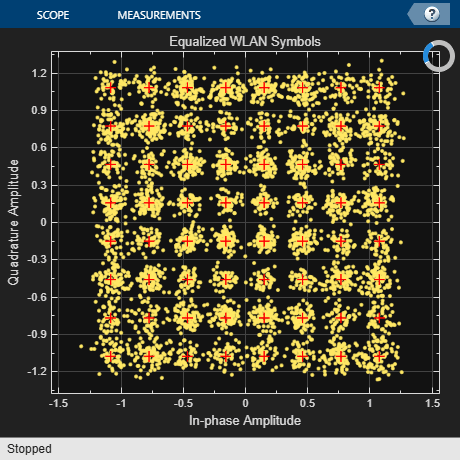``` MAC FCS check pass ```

If using an SDR, release the `sdrTransmitter` and `sdrReceiver` objects to stop the continuous transmission of the 802.11 waveform and to allow for any modification of the SDR object properties.

```if strcmpi(channel,"OverTheAir") release(sdrTransmitter); release(sdrReceiver); end```

Reconstruct Image

Reconstruct the image using the received MAC frames.

```if ~(isempty(fineTimingOffset) || isempty(pktOffset)) % Convert decoded bits from cell array to column vector rxData = cat(1,rxBit{:}); % Remove any extra bits rxData = rxData(1:end-(mod(length(rxData),msduLength*8))); % Reshape such that each column length has bits equal to msduLength*8 rxData = reshape(rxData,msduLength*8,[]); % Remove duplicate packets if any. Duplicate packets are located at the % end of rxData if length(packetSeq)>numMSDUs numDupPackets = size(rxData,2)-numMSDUs; rxData = rxData(:,1:end-numDupPackets); end % Initialize variables for while loop startSeq = []; i=-1; % Only execute this if one of the packet sequence values have been decoded % accurately if any(packetSeq<numMSDUs) while isempty(startSeq) % This searches for a known packetSeq value i = i + 1; startSeq = find(packetSeq==i); end % Circularly shift data so that received packets are in order for image reconstruction. It % is assumed that all packets following the starting packet are received in % order as this is how the image is transmitted. rxData = circshift(rxData,[0 -(startSeq(1)-i-1)]); % Order MAC fragments % Perform bit error rate (BER) calculation on reordered data bitErrorRate = comm.ErrorRate; err = bitErrorRate(double(rxData(:)), ... txDataBits(1:length(reshape(rxData,[],1)))); fprintf(' \nBit Error Rate (BER):\n'); fprintf(' Bit Error Rate (BER) = %0.5f\n',err(1)); fprintf(' Number of bit errors = %d\n',err(2)); fprintf(' Number of transmitted bits = %d\n\n',length(txDataBits)); end decData = bit2int(reshape(rxData(:),8,[]),8,false)'; % Append NaNs to fill any missing image data if length(decData)<length(txImage) numMissingData = length(txImage)-length(decData); decData = [decData;NaN(numMissingData,1)]; else decData = decData(1:length(txImage)); end % Recreate image from received data fprintf('\nConstructing image from received data.\n'); receivedImage = uint8(reshape(decData,imsize)); % Plot received image if exist('imFig','var') && ishandle(imFig) % If Tx figure is open figure(imFig); subplot(212); else figure; subplot(212); end imshow(receivedImage); title(sprintf('Received Image')); end```
``` Bit Error Rate (BER): ```
``` Bit Error Rate (BER) = 0.00000 ```
``` Number of bit errors = 0 ```
``` Number of transmitted bits = 202752 ```
```Constructing image from received data. ```### Further Exploration

• If using an SDR, modify `txGain` or `rxGain` to observe the difference in the EVM and BER after signal reception and processing. You may see errors in the displayed, received image.

• If running the example without an SDR, modify `SNR` to observe the difference in the EVM and BER after signal reception and processing.

• Increase the scaling factor (`scale`) to improve the quality of the received image by generating more transmit bits. This also increases the number of transmitted PPDUs.

• Decrease MSDU size (`msduLength`) to decrease the number of bits transmitted per packet and observe the differences in EVM and BER when a signal is received with a lower SNR.